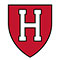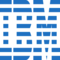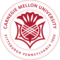Outliers: Definition and Examples in Python

# Outliers: Definition and Examples in Python

clickHere
Updated on Jun 17, 2022 10:49 IST

## Introduction

From the above figure, we have:

• The average weight of the first 4 kids were only 38.75 kg
• The average weight of all kids are 91 kg

Adding the weight of 300 kg shoots the average weight from 38.75 kg to 91 kg.

In statistics, this is called an Outlier.

## Outlier

An outlier is a value in the data set that is extremely distinct from most of the other values.

Let’s understand the outliers by an example of cricket

Highest Batting average of players in Test Cricket:

In the above table, the batting average of the DG Bradman is 99.64, while the batting averages of all remaining is between 54-62.

So, the batting average of DG Bradman is an outlier in the above list.

An outlier in the data is due to

• Variability in the data
• Experimental Error
• Heavy skewness in data
• Missing values

## Detecting Outliers:

• Box-Plot
• Inter-quartile Range
• Z-score

## Box-Plot

It is one of the data visualization methods, where the data is distributed on a box and whisker (also known as box-whisker-plot).

• Data points are divided into 4 different quartiles.
• Box-plot marks Maximum, Minimum, lower quartile (Q1), median (Q2) and upper quartile (Q3).
• Points outside the whisker are Outlier.

Let’s understand by an example in python:

Note: in this article, we are using the insurance.csv file for all of our examples.

Data has 7 features age, sex, BMI, number of children, smoker, region and their expenses of 1338 people.

Dataset is present on Kaggle.

` `
`# import library:import numpy as npimport pandas as pdimport matplotlib.pyplot as pltimport seaborn as sns # import sales datainsurance = pd.read_csv('insurance.csv')insurance #used to get summary statisticsinsurance.describe() #plotting the figureplt.figure(figsize = (4,8))sns.boxplot(y = insurance.bmi)Copy code`

The value lies extremely outside the whisker of the box plot which is an outlier.

## Interquartile Range (IQR):

All the data points that are above and below 1.5 times of Interquartile Range is considered as an outlier.

Steps to find outcome using IQR:

1. Arrange the data orderly (ascending)
2. Compute IQR = Q3 – Q1
3. Calculate bound (upper and lower) 1.5 IQR
4. Any point outside the upper and lower bound are the outlier.

Let’s understand by an example:

` `
`# import library:import numpy as npimport pandas as pdimport matplotlib.pyplot as pltimport seaborn as sns # import sales datainsurance = pd.read_csv('insurance.csv')insurance # defining the outlier function for interquartile rangedef outlier_IQR(df, column):    global lower, upper    Q1 = np.quantile(df[column], 0.25) # first quartile    Q3 = np.quantile(df[column], 0.75) # third quartile    IQR = Q3 - Q1 # inter - quartile range    threshold = 1.5 * IQR # defining the threshold    lower = Q1 - threshold     upper = Q3 + threshold    lower_bound = df[df[column] < lower]    upper_bound = df[df[column] > upper]     #printing IQR, threshold, lower bound, upper bound and total number of outlier     print('IQR is:', IQR)    print('Threshold is:', threshold)    print('Lower bound is:', lower)    print('Upper bound is:', upper)    return print('total number of outliers are:', lower_bound.shape + upper_bound.shape) # getting the IQR, threshold, lower bound, upper bound, total outliersoutlier_IQR(insurance, 'bmi') # plotting figureplt.figure(figsize = (10,8))sns.distplot(insurance.bmi, bins = 25) # creting band to identify the outliers# plt.axvspan : function sets the vertical rectangle across the axes of the plotplt.axvspan(xmin = lower, xmax = insurance.bmi.min(), alpha = 0.2, color = 'red')plt.axvspan(xmin = upper, xmax = insurance.bmi.max(), alpha = 0.2, color = 'red')Copy code`

The red strip on both the sides of the curve indicates the outliers in bmi.

## Z-score:

In a normal distribution, any data point whose z-score is outside the 3rd standard deviation is an outlier.

## Z-score formula:

To know more about Normal Distribution and z-score, read the article Probability Distribution and z-test.

Let’s understand by an example:

` `
`# import library:import numpy as npimport pandas as pdimport matplotlib.pyplot as pltimport seaborn as sns # import sales datainsurance = pd.read_csv('insurance.csv')insurance # defining the outlier function for interquartile rangedef outlier_zscore(df_column):    global outlier, z_score    outlier = []    z_score = []    threshold = 3    mean = np.mean(df_column)    std = np.std(df_column)    for i in df_column:        zscore = (i - mean)/std        z_score.append(zscore)        if np.abs(zscore) > threshold:            outlier.append(i)    return print("total number of outliers", len(outlier)) # getting total number of outlieroutlier_zscore(insurance.bmi) # plotting figureplt.figure(figsize = (10,5))sns.distplot(z_score) # creting band to identify the outliers# plt.axvspan : function sets the vertical rectangle across the axes of the plotplt.axvspan(xmin = 3 ,xmax = max(z_score),alpha = 0.25, color ='red')Copy code`

The red strip on the right side of the curve indicates the outlier in bmi of the insurance data.

To know more about Matplotlib and seaborn, read the article on Matplotlib Pie Chart.

## Conclusion

In this article we will discuss how to detect the outlier in the dataset using Boxplot, IQR and z-score.

clickHere

Vikram has a Postgraduate degree in Applied Mathematics, with a keen interest in Data Science and Machine Learning. He has experience of 2+ years in content creation in Mathematics, Statistics, Data Science, and Mac... Read Full Bio

## Trending Data Science CoursesMachine Learning
Coursera4.6## Top Picks & New Arrivals